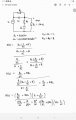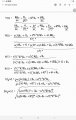# Finding correct value of components to get the centre frequency of a band reject filter at 30kHz

#### zuhriri

Joined Jan 1, 2021
4
Hello!

I have to design a band reject filter using two capacitors, two resistors and a load resistor of 1kOhm.
I already figured out the appropriate circuit and had derive the transfer function of the filter.I had chose the values for the capacitors, C = 0.01uF and the value of load resistor is 1kOhm.
Now I want to find the values of R, when the centre frequency is 30kHz/60000pi rads-1.

To find the R, all I need to do is substitute the value of C, RL, omega=60000pi and the magnitude |H(s)| = 0.

The problem is I obtained the values of R, and when I simulate the circuit, the centre frequency is not at 30kHz(I've tried all the values too)

I also checked the transfer function derived a few times and so far found no mistakes, I think.

So, do anyone have any idea why is the obtained values of R is incorrect? Maybe I might have missed a few important points.

#### Papabravo

Joined Feb 24, 2006
20,603
Are you convinced that the solution is unique? Could their be more than one solution?
In passive circuits it is not uncommon for the impedance of the 2nd stage to load down the first stage, shifting the response.
This tends not to happen in an active filter because the opamp isolates the stages.
You should realize that the 2nd stage of the filter is in parallel with the 1KΩ resistor, so the impedance seen by the first stage is a maximum of 1KΩ. This creates a significant load.

#### zuhriri

Joined Jan 1, 2021
4
Are you convinced that the solution is unique? Could their be more than one solution?
The solution is not unique, I believe that there are like 4 solutions(I may be wrong), and I've tried them all. Unfortunately, I am unable to share the solutions since I'm not at home now. But I guess as soon I get home, I'll try again. However, my transfer function is correct right?

#### Papabravo

Joined Feb 24, 2006
20,603
In fact the number of solutions is significantly larger.
See edit to post #2

#### MrAl

Joined Jun 17, 2014
10,904
The solution is not unique, I believe that there are like 4 solutions(I may be wrong), and I've tried them all. Unfortunately, I am unable to share the solutions since I'm not at home now. But I guess as soon I get home, I'll try again. However, my transfer function is correct right?
If you assume R=R1=R2 and C=C1=C2 and RL is fixed and you choose some C also fixed then yes there are four solutions for R but probably only one of them is a 'real life' solution that is not imaginary and not negative (positive real).

Not sure what you did so far really and why dont you post the value you got for R so we can see what went wrong.

The solution with the above assumptions and RL=1k is an optimization problem where you just have to find the min or max of a function. Once you do that, you can solve for R directly but you probably wont get if from just w=1/RC.
What you have to do is write the complex frequency domain equation for the output then take the norm of that (or what we call the magnitude or amplitude). Once you get that, you take the first derivative of that with respect to w and then solve for w to find what w is with given R knowing C and RL, or you solve for R knowing w and C and RL. The result is a formula with w, C, and RL in it.

Did you ever do this kind of problem before?

Assuming you are using 0.01uf for C, the result for R is between 400 and 500 Ohms but no it is not 450 Ohms either.